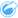# Find Spatial Mean

Find Spatial Mean calculates the geographic center of a set of point features. The output will be a single point feature (or a point feature for each group in the dataset) located at the geographic center or the center of concentration of the points.

## Example

A crime analyst is analyzing the location of crime incidents based on the time of day when the incidents occurred. Comparing the spatial mean of daytime crimes to that of nighttime crimes allows the police department to reallocate their resources more effectively.

## Use the Find Spatial Mean capability

Find Spatial Mean can be run on maps with point layers.

Complete the following steps to run the Find Spatial Mean analysis capability:

1. If necessary, click the map card to activate it. A card is active when the toolbar and Action buttonappear.
2. Click the Action button and choose Find Spatial Mean.
3. For Choose a layer, select the layer for which you want to calculate the spatial mean.
4. Expand Additional options and enter values for the Weight, Group by, and Add statistics to summarize parameters, if necessary.
5. Click Run.

## Usage notes

The Choose a layer parameter is used to select the dataset for which to calculate spatial mean. Only point features are available in the drop-down menu.

You can expand Additional options to reveal the Weight, Group by, and Add statistics to summarize parameters. The following table summarizes these parameters, including their default values:

ParameterDescriptionDefault value

Weight

A number field denoting the value of each feature that is used to calculate a weighted mean based on relative importance. For example, stores in a retail chain can be weighted by total sales.

None. The spatial mean is based only on location.

Group by

A string field used to group features for separate spatial mean calculations based on shared values. A separate spatial mean is calculated for each value in the field. For example, wildlife observations in a national park can be grouped by species to determine optimal locations for sightings.

None. The spatial mean is calculated for all features.

One or more number, rate/ratio, or string fields used to calculate summary statistics for the dataset. For number and rate/ratio fields, the sum, minimum, maximum, average, and mode can be calculated. For string fields, the mode can be calculated.

If a Group by field is used, the summary statistics are calculated separately for each group.

None. The count of features is included in the output dataset.

## Limitations

Find Spatial Mean is not supported for read-only connections to Google BigQuery, Snowflake, and database platforms that are not supported out-of-the-box.

Google BigQuery does not support mode calculations.